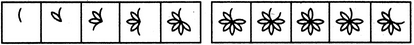# Non Verbal Reasoning - Series

Exercise : Series - Section 2
Directions to Solve

Each of the following questions consists of five figures marked A, B, C, D and E called the Problem Figures followed by five other figures marked 1, 2, 3, 4 and 5 called the Answer Figures. Select a figure from amongst the Answer Figures which will continue the same series as established by the five Problem Figures.

1.

Select a figure from amongst the Answer Figures which will continue the same series as established by the five Problem Figures.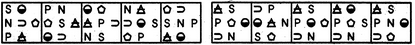(A)     (B)     (C)     (D)     (E)       (1)     (2)     (3)     (4)     (5)
1
2
3
4
5
Explanation:
In each step, all the symbols move in the sequence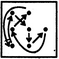2.

Select a figure from amongst the Answer Figures which will continue the same series as established by the five Problem Figures.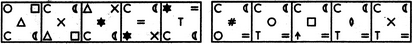(A)     (B)     (C)     (D)     (E)       (1)     (2)     (3)     (4)     (5)
1
2
3
4
5
Explanation:
The symbols move in the sequences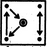and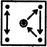alternately. In each step, the symbol that reaches the-encircled position gets replaced by a new symbol.

3.

Select a figure from amongst the Answer Figures which will continue the same series as established by the five Problem Figures.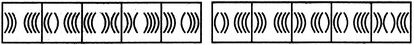(A)     (B)     (C)     (D)     (E)       (1)     (2)     (3)     (4)     (5)
1
2
3
4
5
Explanation:
Two, three, four, two, three,..... curves get inverted sequentially.

4.

Select a figure from amongst the Answer Figures which will continue the same series as established by the five Problem Figures.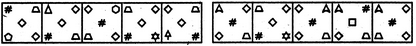(A)     (B)     (C)     (D)     (E)       (1)     (2)     (3)     (4)     (5)
1
2
3
4
5
Explanation:
The symbols move in the sequence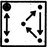in the first step. In each subsequent step, the symbols move in the sequence obtained by rotating the previous sequence through 90oCW. Also, in each step, the symbol that reaches the encircled position gets replaced by a new one.

5.

Select a figure from amongst the Answer Figures which will continue the same series as established by the five Problem Figures.